# Question no 1 A] How many electrons are necessary to produce 1.2 C of negative charge?...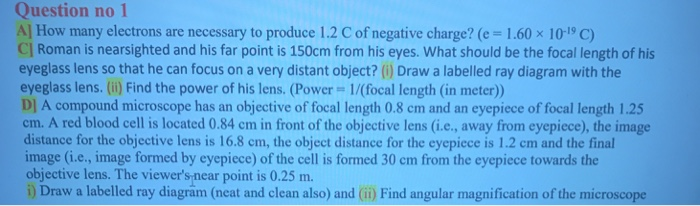Question no 1 A] How many electrons are necessary to produce 1.2 C of negative charge? (e 1.60 x 10-19 C) CI Roman is nearsighted and his far point is 150cm from his eyes. What should be the focal length of his eyeglass lens so that he can focus on a very distant object? (i) Draw a labelled ray diagram with the eyeglass lens. (i) Find the power of his lens. (Power- 1/(focal length (in meter) D] A compound microscope has an objective of focal length 0.8 cm and an eyepiece of focal length 1.25 cm. A red blood cell is located 0.84 cm in front of the objective lens (i.e., away from eyepiece), the image distance for the objective lens is 16.8 cm, the object distance for the eyepiece is 1.2 cm and the final image (i.e., image formed by eyepiece) of the cell is formed 30 cm from the eyepiece towards the objective lens. The viewer's near point is 0.25 m. i) Draw a labelled ray diagram (neat and clean also) and (i) Find angular magnification of the microscope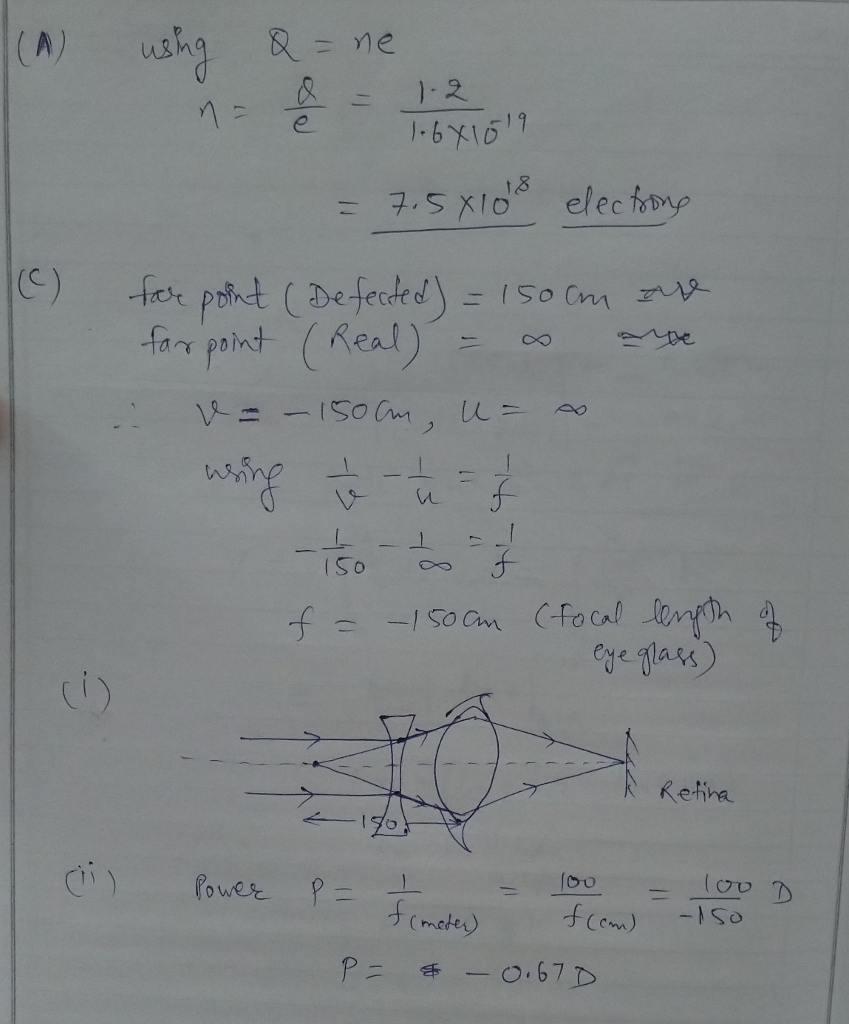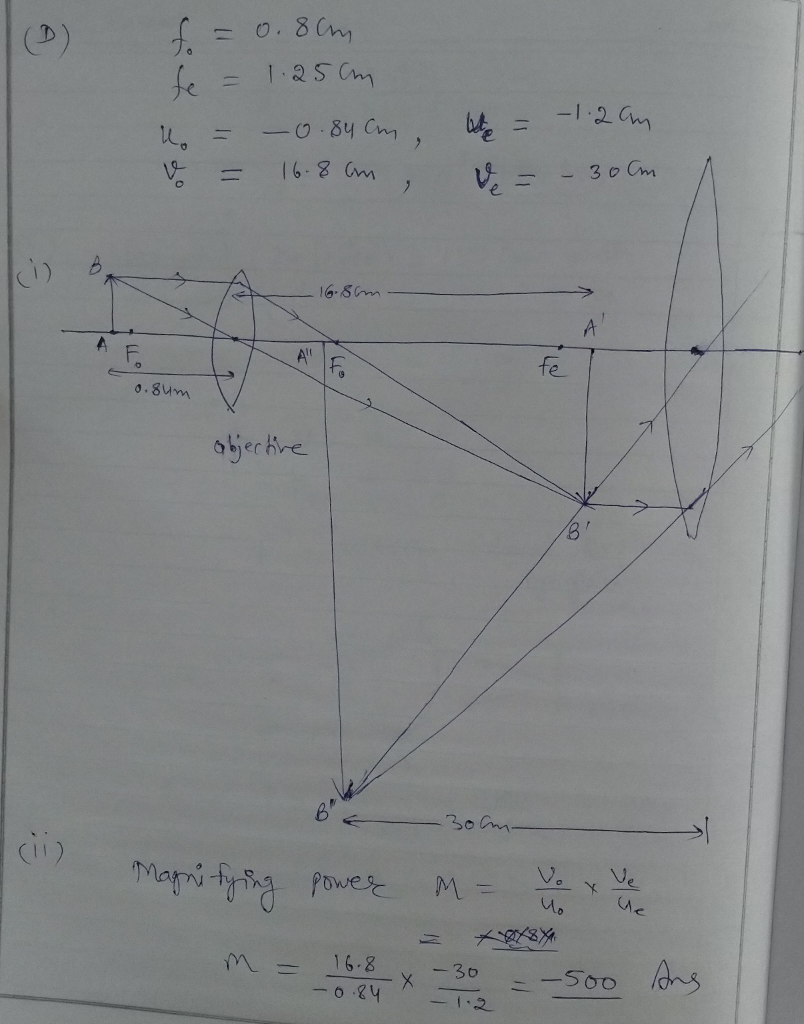#### Earn Coin

Coins can be redeemed for fabulous gifts.

Similar Homework Help Questions
• ### A microscope has an objective lens with a focal length of 19.5 mm and an eyepiece with a focal length of 6.2 mm. With the length of the barrel set an 33.0 cm, the diameter of a red blood cell’s image...

A microscope has an objective lens with a focal length of 19.5 mm and an eyepiece with a focal length of 6.2 mm. With the length of the barrel set an 33.0 cm, the diameter of a red blood cell’s image subtends an angle of 1.2 milli-radians with the eye. The final image distance is 29.0 cm from the eyepiece. (a) What is the length of the image of the of the cell produced by the eyepiece? (b) Using the...

• ### please I need answers to all questions because am writing text on Friday. 7. Suppose an...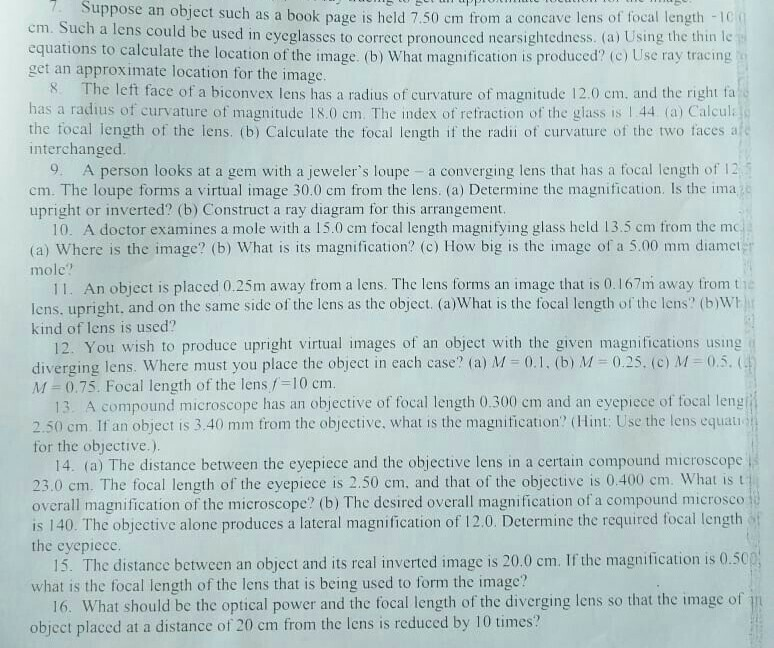please I need answers to all questions because am writing text on Friday. 7. Suppose an object such as a book page is held 7.50 cm from a concave lens of focal length -1C em. Such a lens could be used in eyeglasses to correct pronounced nearsightedness. (a) Using the thin le equations to calculate the location of the image. (b) What magnification is produced? (c) Use ray tracing get an approximate location for the image. 8. The left face...

• ### A Galilean telescope is made with a 40 cm focal length objective lens and a -10 cm focal length e...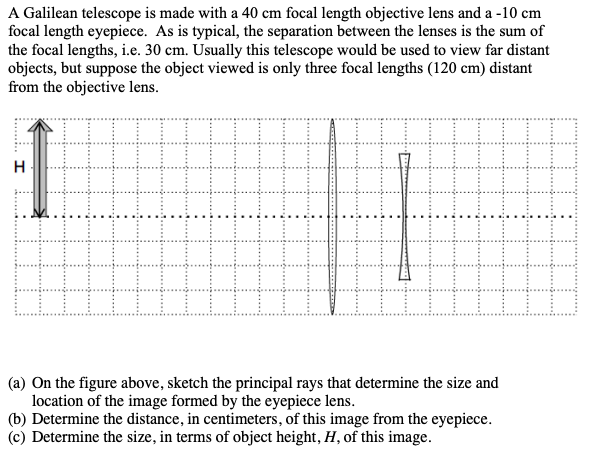A Galilean telescope is made with a 40 cm focal length objective lens and a -10 cm focal length eyepiece. As is typical, the separation between the lenses is the sum of the focal lengths, i.e. 30 cm. Usually this telescope would be used to view far distant objects, but suppose the object viewed is only three focal lengths (120 cm) distant from the objective lens (a) On the figure above, sketch the principal rays that determine the size and...

• ### A Galilean telescope is made with a 40 cm focal length objective lens and a -10 cm focal length e...A Galilean telescope is made with a 40 cm focal length objective lens and a -10 cm focal length eyepiece. As is typical, the separation between the lenses is the sum of the focal lengths, i.e. 30 cm. Usually this telescope would be used to view far distant objects, but suppose the object viewed is only three focal lengths (120 cm) distant from the objective lens (a) On the figure above, sketch the principal rays that determine the size and...

• ### 90 cm, an eyepiece lens with a fosal length of 6.20 m and a barrel A custom-made compound microcp...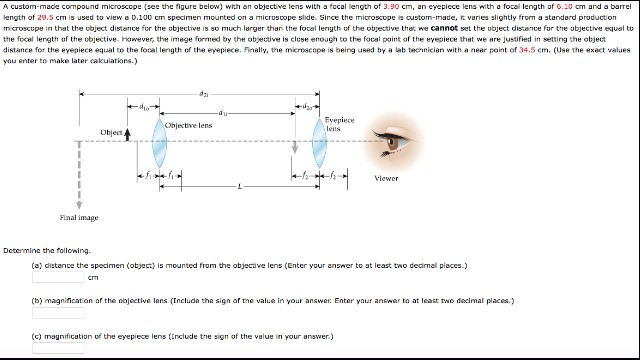90 cm, an eyepiece lens with a fosal length of 6.20 m and a barrel A custom-made compound microcpe (see the figure below) wih an objective lens with a focal length of 3 ength of 29.5 cm is used to view a D.100 cm specimen mounted on a microscope slide. Since the miaroscope is custom-made, varies slightly from a standard production rn croscope in that the object distance for the ubjectve ฯ Bo much larger than the otel length of...

• ### 2) A schematic diagram of a refractive microscope is shown in Figure 1. The focal length...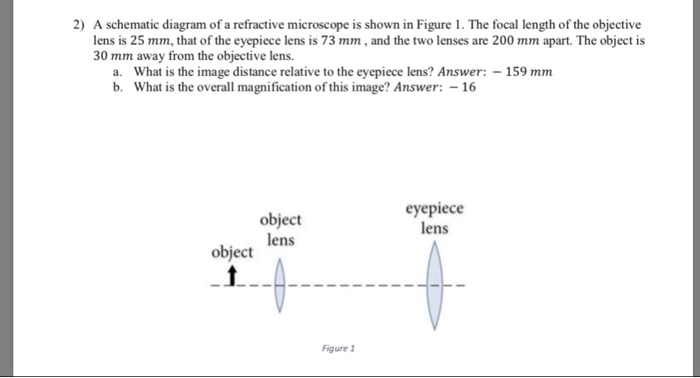2) A schematic diagram of a refractive microscope is shown in Figure 1. The focal length of the objective lens is 25 mm, that of the eyepiece lens is 73 mm, and the two lenses are 200 mm apart. The object is 30 mm away from the objective lens. a. What is the image distance relative to the eyepiece lens? Answer: b. What is the overall magnification of this image? Answer:-16 159 mm eyepiece lens object lens object Figure

• ### A. How far from the objective lens is the first image formed? B. What is the...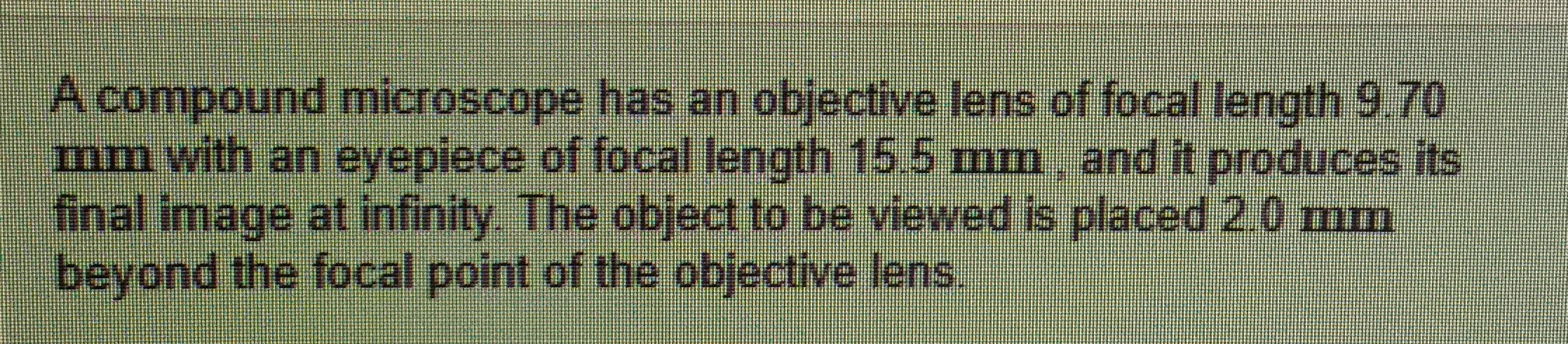A. How far from the objective lens is the first image formed? B. What is the overall magnification of this microscope? A compound microscope has an objective lens of focal length 9.70 mm with an eyepiece of focal length 15.5 mm, and it produces its final image at infinity. The object to be viewed is placed 2.0 mm beyond the focal point of the objective lens

• ### Experiment 22: The Compound Microscope f2 Object 2 1 (Objective Lens)d L, (Eyepiece) d#150mm The Compound Microsco...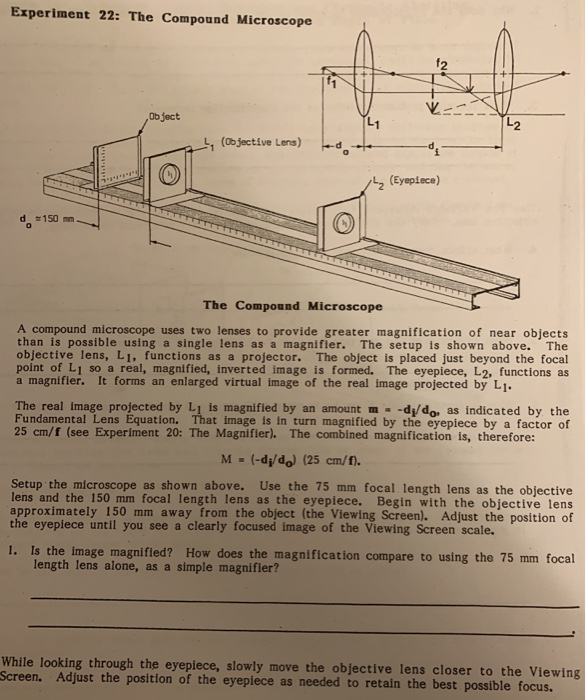Experiment 22: The Compound Microscope f2 Object 2 1 (Objective Lens)d L, (Eyepiece) d#150mm The Compound Microscope A compound microscope uses two lenses to provide greater magnification of near objects than is possible using a single lens as a magnifier. The setup is shown above. The objective lens, L1, functions as a projector. The object is placed just beyond the focal point of L1 so a real, magnified, inverted image is formed. The eyepiece, L2, functions as a magnifier. It...

• ### 4 of 5 The focal length of the eyepiece of a certain microscope is 20.0 mm....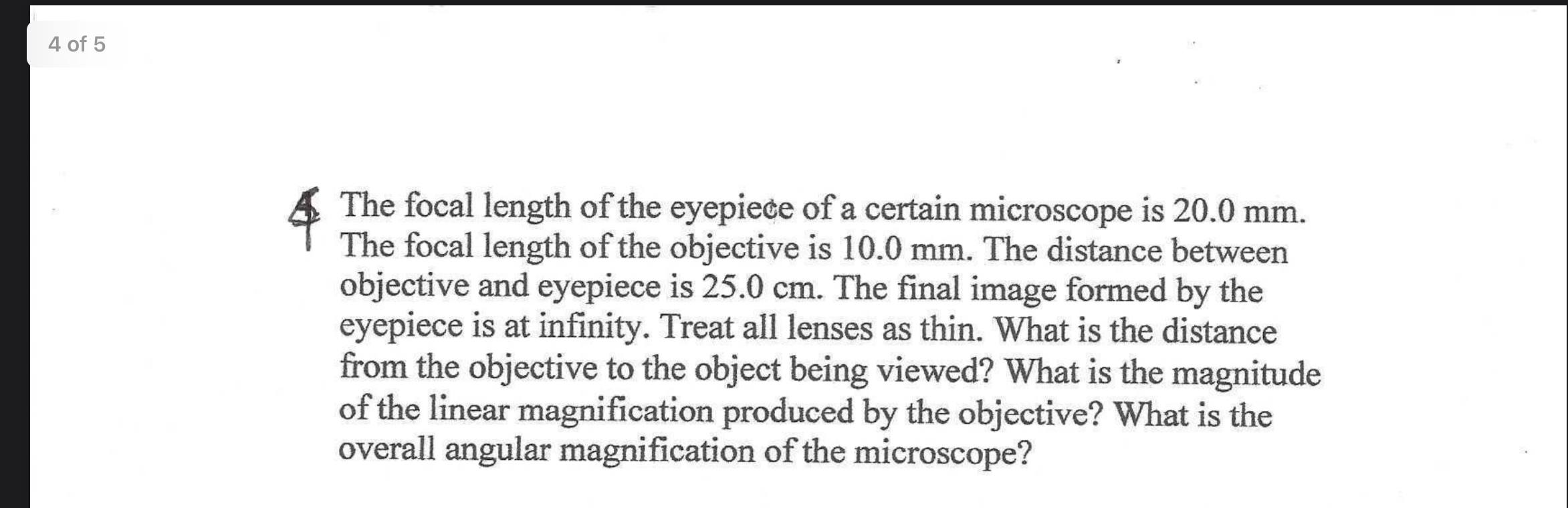4 of 5 The focal length of the eyepiece of a certain microscope is 20.0 mm. The focal length of the objective is 10.0 mm. The distance between objective and eyepiece is 25.0 cm. The final image formed by the eyepiece is at infinity. Treat all lenses as thin. What is the distance from the objective to the object being viewed? What is the magnitude of the linear magnification produced by the objective? What is the overall angular magnification of...

• ### In a two-lens system, the image produced by one lens acts as the object for the next lens. This simple principle finds...

In a two-lens system, the image produced by one lens acts as the object for the next lens. This simple principle finds applications in many optical instruments, including some of common use such as the microscope and the telescope. The microscope available in your biology lab has a converging lens (the eyepiece) with a focal length of 2.50 cm mounted on one end of a tube of adjustable length. At the other end is another converging lens (the objective) that...

Free Homework App